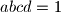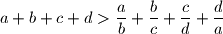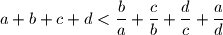### IMO Shortlist 2008 problem A5

Kvaliteta:
Avg: 3,0
Težina:
Avg: 7,7
Let$a$,$b$,$c$,$d$ be positive real numbers such that$abcd = 1$ and$a + b + c + d > \dfrac{a}{b} + \dfrac{b}{c} + \dfrac{c}{d} + \dfrac{d}{a}$. Prove thatProposed by Pavel Novotný, Slovakia
Izvor: Međunarodna matematička olimpijada, shortlist 2008